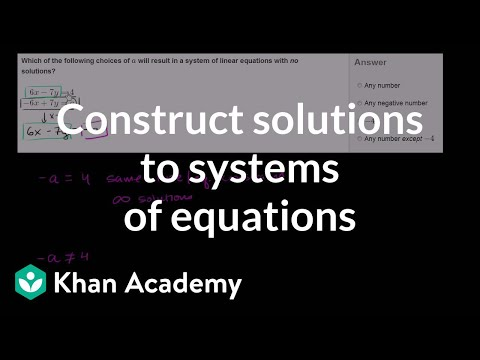# Intercepts from an equation (Full video)

Description: Sal finds the x and y-intercepts of -5x + 4y = 20. Created by Sal Khan and Monterey Institute for Technology and Education. We have the equation negative 5x plus 4y is equal to 20, and we're told to find the intercepts of this equation. The x-intercept is when y is equal to 0, right? Well, I'm not to the right or the left, so my x value has to be 0, so the y-intercept occurs when x is equal to 0.

### Other videos you might be interested in### Completing solutions to 2-variable equations (Full video)